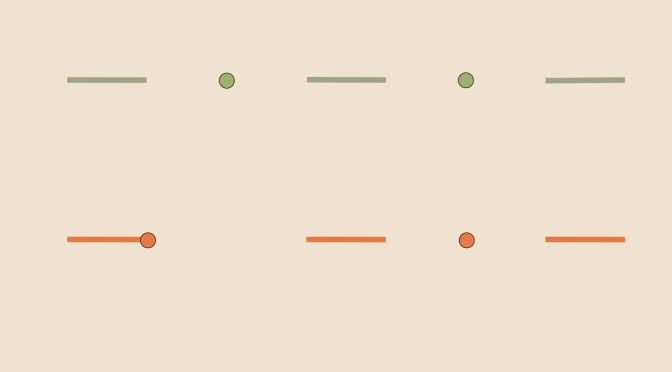Two continuous bijections but no homeomorphism

We consider here two topological spaces $$X$$ and $$Y$$. We also suppose that $$f$$ is a continuous bijection from $$X$$ to $$Y$$ and $$g$$ a continuous bijection from $$Y$$ to $$X$$. Providing such hypothesis (H), is there necessarily an homeomorphism from $$X$$ to $$Y$$?

We first look at the simple case where $$X$$ is an interval $$I$$ equipped with the subspace topology. If $$f$$ is a continuous map defined from $$I$$ to $$\mathbb{R}$$ and $$f$$ is supposed to be bijective, $$f$$ is therefore one-to-one. According to a well known result, $$f$$ is strictly monotonic on $$I$$, the image of $$I$$ under $$f$$ is an interval $$J$$ and the inverse map $$f^{-1}$$ is continuous. Hence, if $$X$$ and $$Y$$ are two intervals, an homomorphism always exists providing the hypothesis (H).

For the second case, we consider a compact space $$X$$ and a Haussdorf space $$Y$$. Suppose that $$f$$ is a continuous bijection from $$X$$ to $$Y$$. Then $$Y$$ is compact and $$f$$ is an homeomorphism. For the prove we need to show that $$f^{-1}$$ is continuous. For this, consider any closed subspace $$F \subset X$$. $$F$$ is compact and the inverse image of $$F$$ by $$f^{-1}$$ is $$f(F)$$, which is compact and hence closed. Finally, $$f^{-1}$$ is continuous and $$f$$ is an homeomorphism.

Finally we give a counterexample of two topological spaces $$X$$ and $$Y$$ which satisfy the hypothesis (H) while no homomorphism exists from $$X$$ to $$Y$$. We take as spaces $$\displaystyle X=\bigcup_{n=0}^\infty ((3n,3n+1) \cup \{3n+2\})$$ and $$Y=(X \setminus \{2\}) \cup \{1\}$$. And for the continuous bijections $$f: X \rightarrow Y$$ defined by:
$f(x)=\begin{cases} x & \text{if } x \neq 2\\ 1 & \text{if } x = 2\\ \end{cases}$ and $$g: Y \rightarrow X$$ defined by:
$g(y)=\begin{cases} y/2 & \text{if } y \le 1\\ y/2 – 1 & \text{if } 3 < y <4\\ y-3 & \text{if } y \ge 5\\ \end{cases}$ The continuity of $$f$$ is clear at any point $$x \in X \setminus \{2\}$$. $$f$$ is also continuous at $$2$$ as for $$\vert x-2 \vert < 1/2$$ and $$x \in X$$ we have $$f(x)=1$$. One can also prove that $$g$$ is continuous. Let now suppose that $$h:Y \rightarrow X$$ is an homeomorphism. The image under $$h$$ of the open interval $$(0,1)$$ is an open interval included in an interval $$(3n,3n+1)$$ with $$n \in \mathbb{N}$$. In fact it is even the complete interval $$(3n,3n+1)$$. If it wasn't the case, we would have $$h^{-1}((3n,3n+1) \setminus h((0,1))) \subset [2,+\infty)$$, hence $$h^{-1}$$ wouldn't be continuous. And we get a contradiction as $$h(1)$$ cannot be defined.